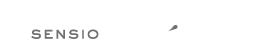# Rule `random_api_migration`¶

Replaces `rand`, `srand`, `getrandmax` functions calls with their `mt_*` analogs or `random_int`.

## Warning¶

### Using this rule is risky¶

Risky when the configured functions are overridden. Or when relying on the seed based generating of the numbers.

## Configuration¶

### `replacements`¶

Mapping between replaced functions with the new ones.

Allowed types: `array`

Default value: `['getrandmax' => 'mt_getrandmax', 'rand' => 'mt_rand', 'srand' => 'mt_srand']`

## Examples¶

### Example #1¶

Default configuration.

```--- Original
+++ New
<?php
-\$a = getrandmax();
-\$a = rand(\$b, \$c);
-\$a = srand();
+\$a = mt_getrandmax();
+\$a = mt_rand(\$b, \$c);
+\$a = mt_srand();
```

### Example #2¶

With configuration: `['replacements' => ['getrandmax' => 'mt_getrandmax']]`.

```--- Original
+++ New
<?php
-\$a = getrandmax();
+\$a = mt_getrandmax();
\$a = rand(\$b, \$c);
\$a = srand();
```

### Example #3¶

With configuration: `['replacements' => ['rand' => 'random_int']]`.

```--- Original
+++ New
-<?php \$a = rand(\$b, \$c);
+<?php \$a = random_int(\$b, \$c);
```

## Rule sets¶

The rule is part of the following rule sets:

• @PHP70Migration:risky with config:

`['replacements' => ['mt_rand' => 'random_int', 'rand' => 'random_int']]`

• @PHP71Migration:risky with config:

`['replacements' => ['mt_rand' => 'random_int', 'rand' => 'random_int']]`

• @PHP74Migration:risky with config:

`['replacements' => ['mt_rand' => 'random_int', 'rand' => 'random_int']]`

• @PHP80Migration:risky with config:

`['replacements' => ['mt_rand' => 'random_int', 'rand' => 'random_int']]`

The logo is © 2010-2022 Sensio Labs